Скачать презентацию — About the consumers — The plan of

24626cfe3cddf2bd6dfd79a8a34ebebd.ppt

• Количество слайдов: 23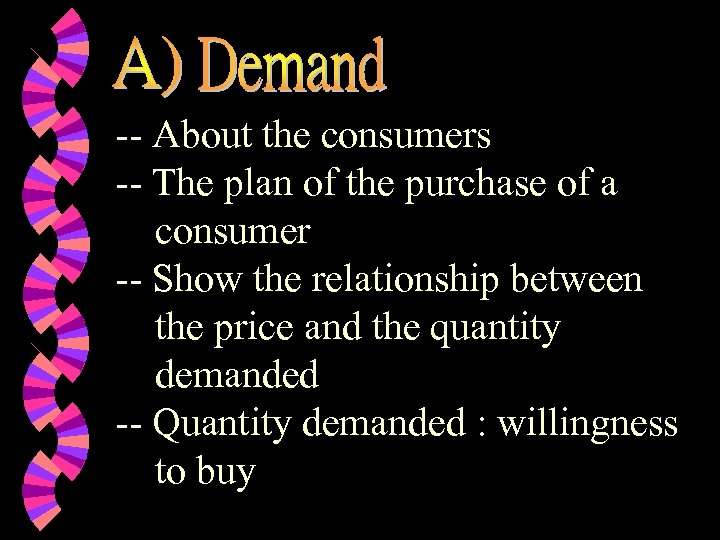-- About the consumers -- The plan of the purchase of a consumer -- Show the relationship between the price and the quantity demanded -- Quantity demanded : willingness to buy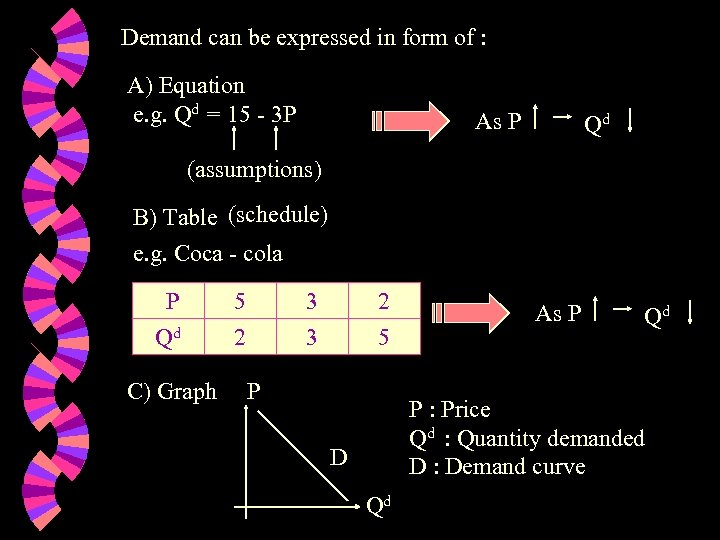Demand can be expressed in form of : A) Equation e. g. Qd = 15 - 3 P As P Qd (assumptions) B) Table (schedule) e. g. Coca - cola PP Qd C) Graph 5 2 3 3 2 5 P As P Qd P : Price Qd : Quantity demanded D : Demand curve D Qd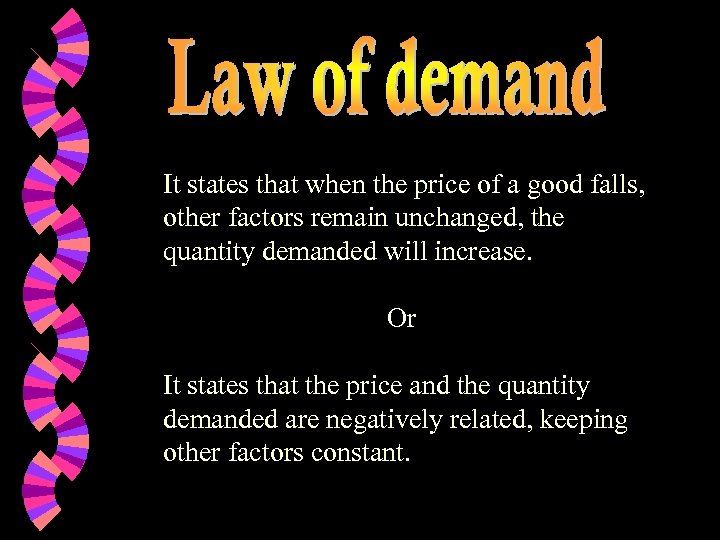It states that when the price of a good falls, other factors remain unchanged, the quantity demanded will increase. Or It states that the price and the quantity demanded are negatively related, keeping other factors constant.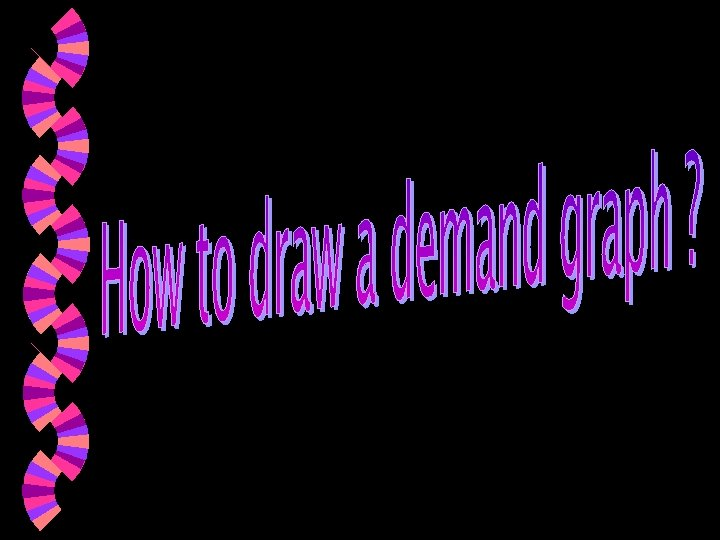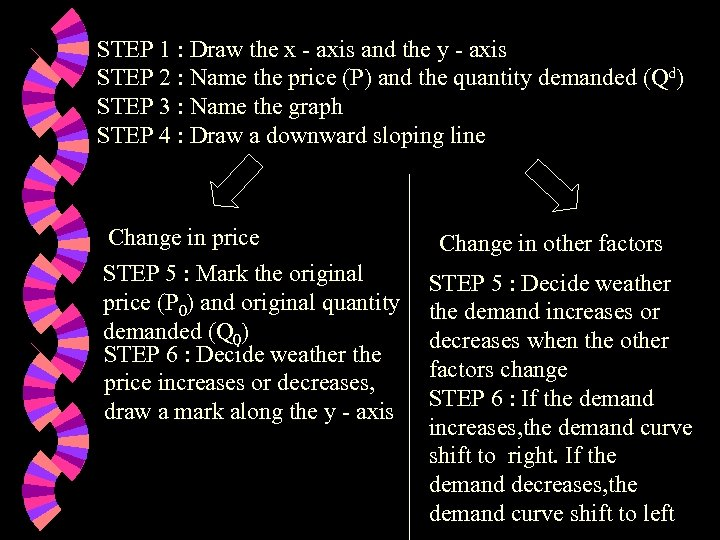STEP 1 : Draw the x - axis and the y - axis STEP 2 : Name the price (P) and the quantity demanded (Qd) STEP 3 : Name the graph STEP 4 : Draw a downward sloping line Change in price STEP 5 : Mark the original price (P 0) and original quantity demanded (Q 0) STEP 6 : Decide weather the price increases or decreases, draw a mark along the y - axis Change in other factors STEP 5 : Decide weather the demand increases or decreases when the other factors change STEP 6 : If the demand increases, the demand curve shift to right. If the demand decreases, the demand curve shift to left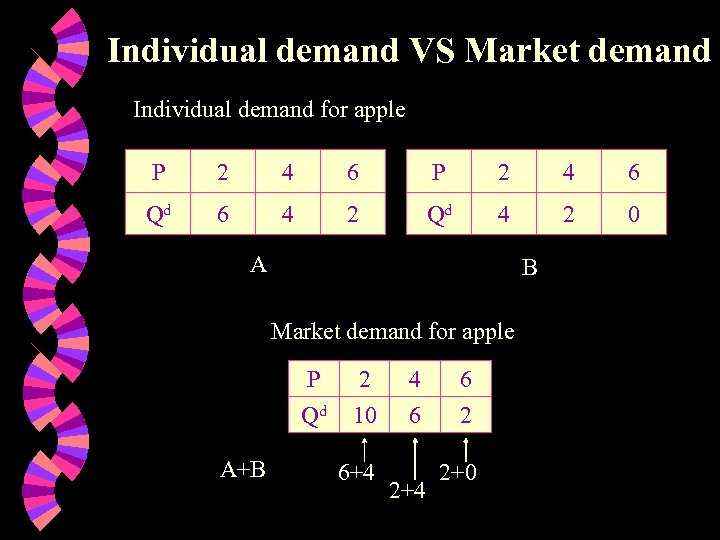Individual demand VS Market demand Individual demand for apple P 2 4 6 Qd 6 4 2 Qd 4 2 0 A B Market demand for apple P Qd A+B 2 10 6+4 4 6 2+4 6 2 2+0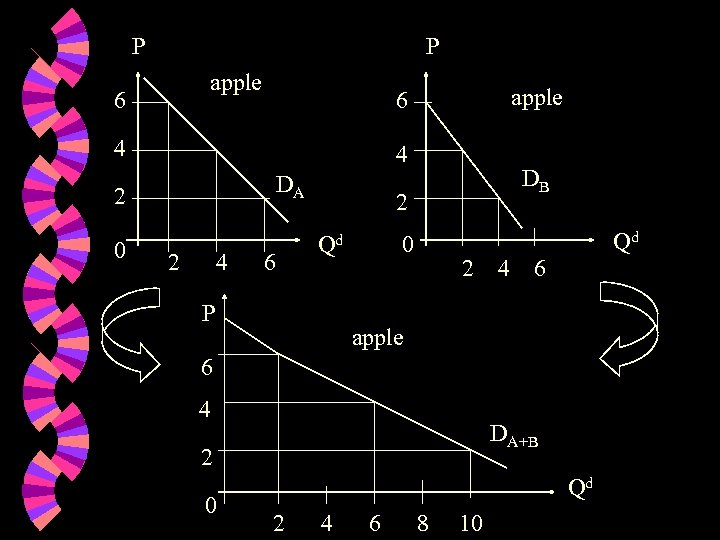P P apple 6 4 4 DA 2 0 apple 6 2 4 6 DB 2 Qd P Qd 0 2 4 6 apple 6 4 DA+B 2 0 Qd 2 4 6 8 10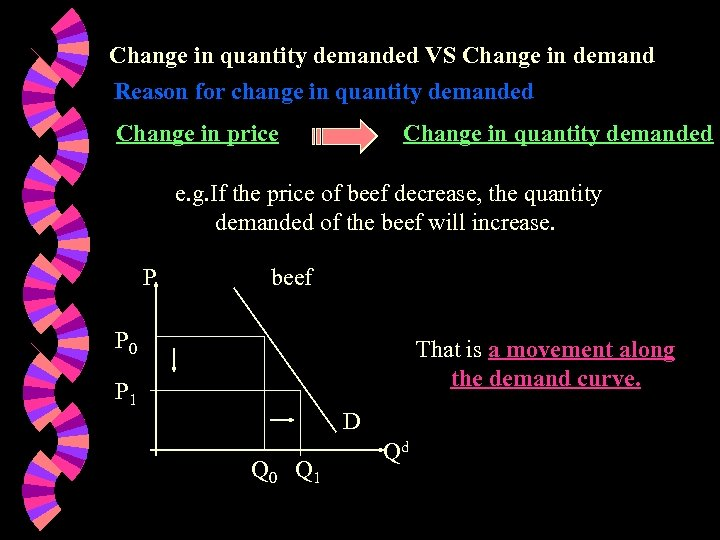Change in quantity demanded VS Change in demand Reason for change in quantity demanded Change in price Change in quantity demanded e. g. If the price of beef decrease, the quantity demanded of the beef will increase. P beef P 0 That is a movement along the demand curve. P 1 D Q 0 Q 1 Qd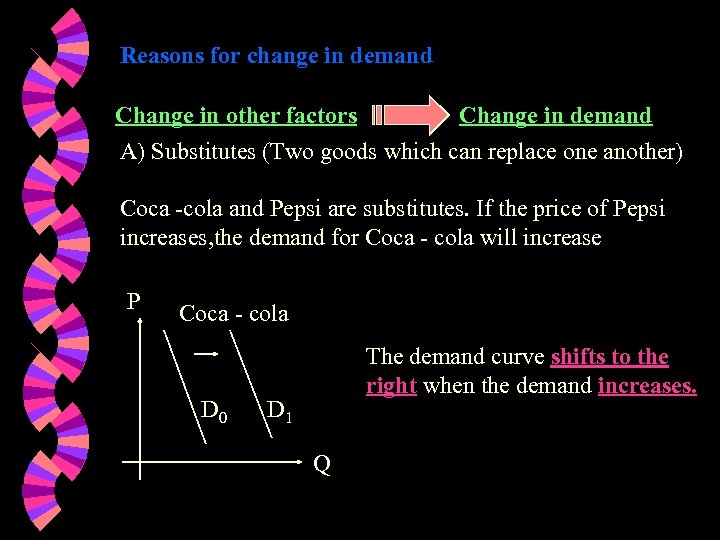Reasons for change in demand Change in other factors Change in demand A) Substitutes (Two goods which can replace one another) Coca -cola and Pepsi are substitutes. If the price of Pepsi increases, the demand for Coca - cola will increase P Coca - cola D 0 The demand curve shifts to the right when the demand increases. D 1 Q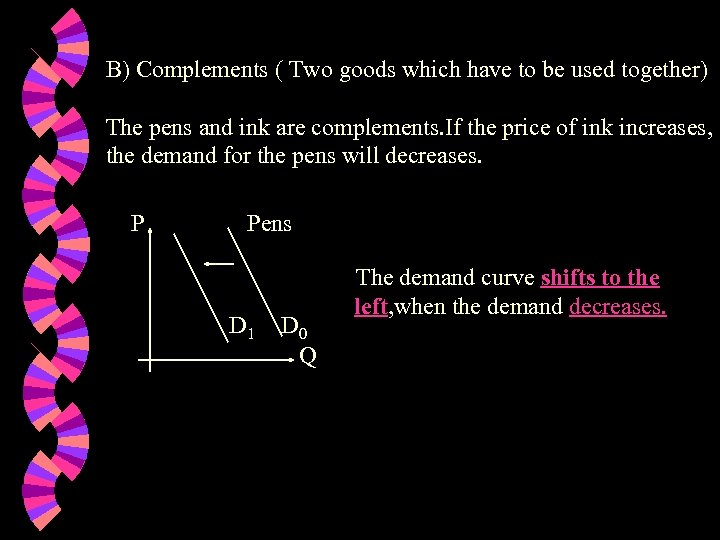B) Complements ( Two goods which have to be used together) The pens and ink are complements. If the price of ink increases, the demand for the pens will decreases. P Pens D 1 D 0 Q The demand curve shifts to the left, when the demand decreases.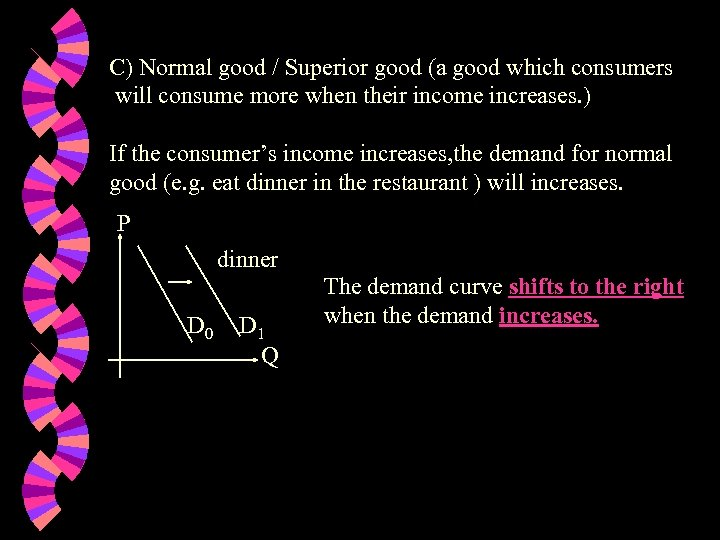C) Normal good / Superior good (a good which consumers will consume more when their income increases. ) If the consumer’s income increases, the demand for normal good (e. g. eat dinner in the restaurant ) will increases. P dinner D 0 D 1 Q The demand curve shifts to the right when the demand increases.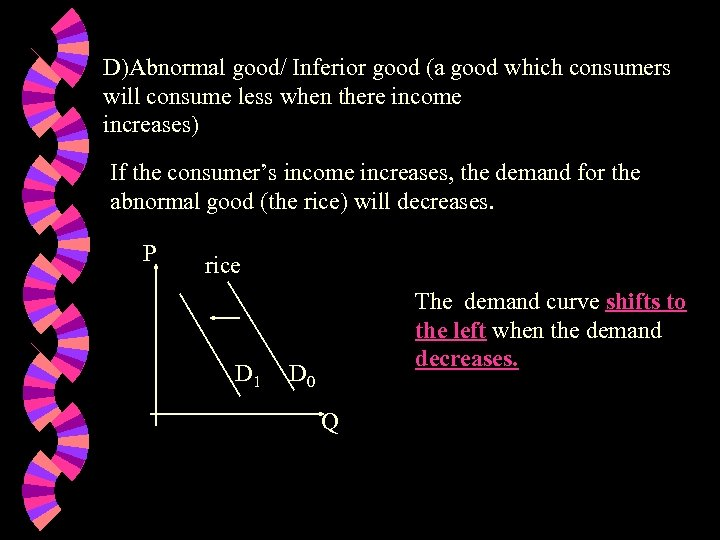D)Abnormal good/ Inferior good (a good which consumers will consume less when there income increases) If the consumer’s income increases, the demand for the abnormal good (the rice) will decreases. P rice D 1 The demand curve shifts to the left when the demand decreases. D 0 Q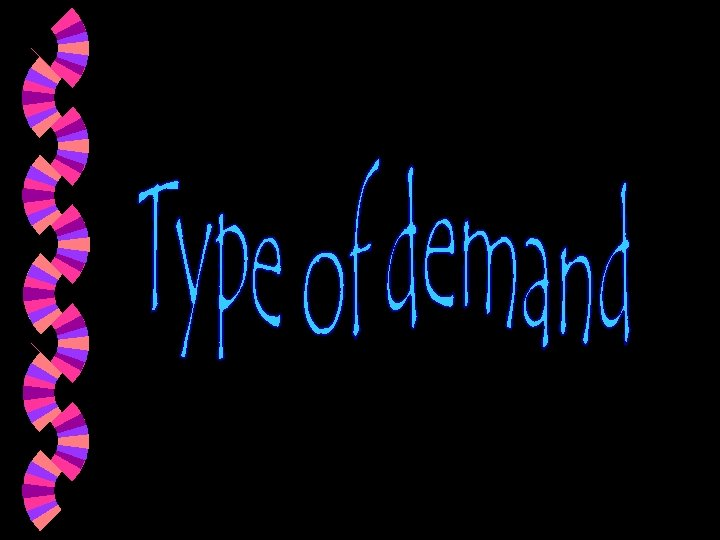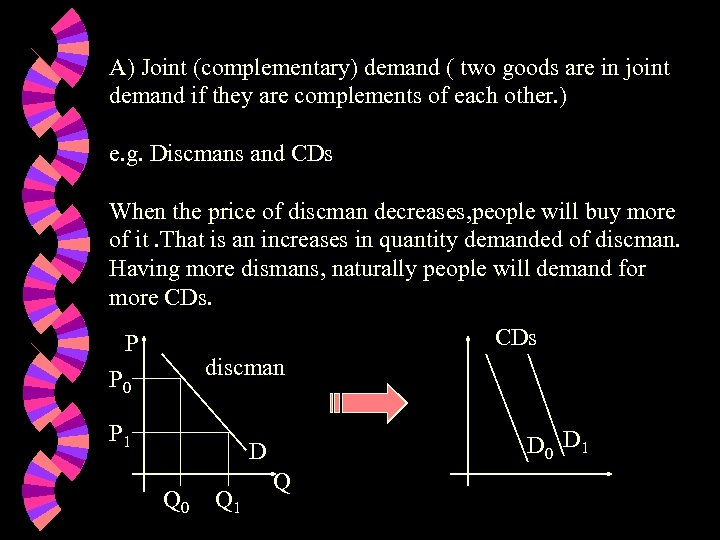A) Joint (complementary) demand ( two goods are in joint demand if they are complements of each other. ) e. g. Discmans and CDs When the price of discman decreases, people will buy more of it. That is an increases in quantity demanded of discman. Having more dismans, naturally people will demand for more CDs P discman P 0 P 1 D 0 D 1 D Q 0 Q 1 Q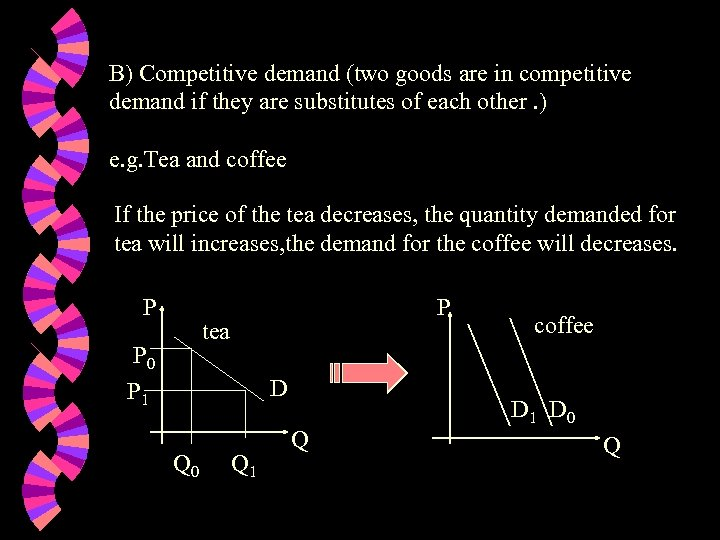B) Competitive demand (two goods are in competitive demand if they are substitutes of each other. ) e. g. Tea and coffee If the price of the tea decreases, the quantity demanded for tea will increases, the demand for the coffee will decreases. P P tea P 0 D P 1 Q 0 Q 1 Q coffee D 1 D 0 Q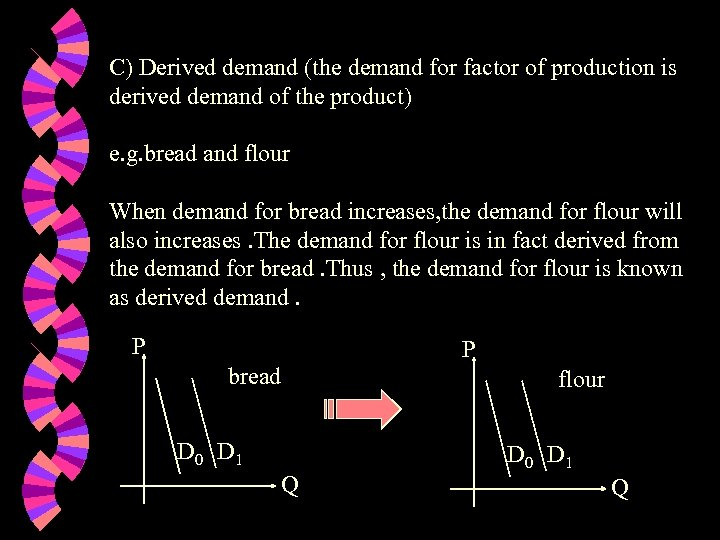C) Derived demand (the demand for factor of production is derived demand of the product) e. g. bread and flour When demand for bread increases, the demand for flour will also increases. The demand for flour is in fact derived from the demand for bread. Thus , the demand for flour is known as derived demand. P P bread flour D 0 D 1 Q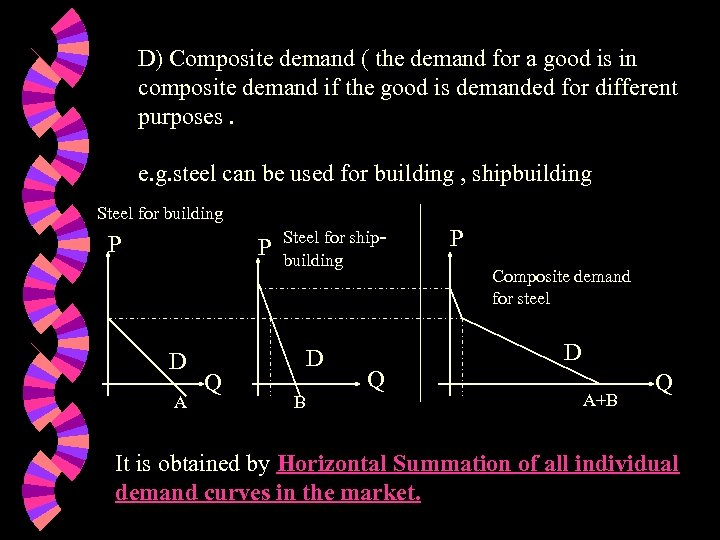D) Composite demand ( the demand for a good is in composite demand if the good is demanded for different purposes. e. g. steel can be used for building , shipbuilding Steel for building P P D A Q Steel for shipbuilding D B P Composite demand for steel D Q A+B Q It is obtained by Horizontal Summation of all individual demand curves in the market.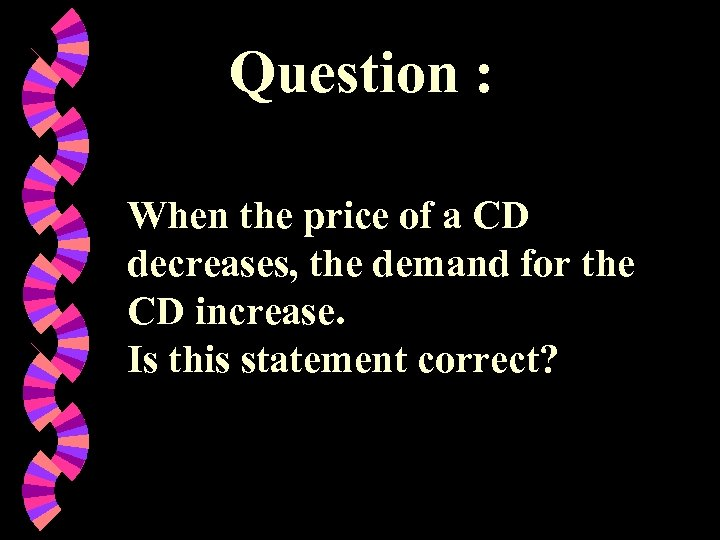Question : When the price of a CD decreases, the demand for the CD increase. Is this statement correct?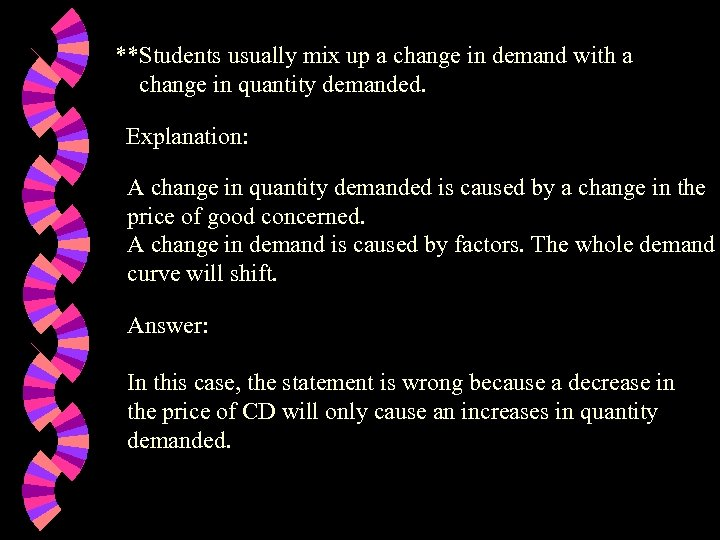**Students usually mix up a change in demand with a change in quantity demanded. Explanation: A change in quantity demanded is caused by a change in the price of good concerned. A change in demand is caused by factors. The whole demand curve will shift. Answer: In this case, the statement is wrong because a decrease in the price of CD will only cause an increases in quantity demanded.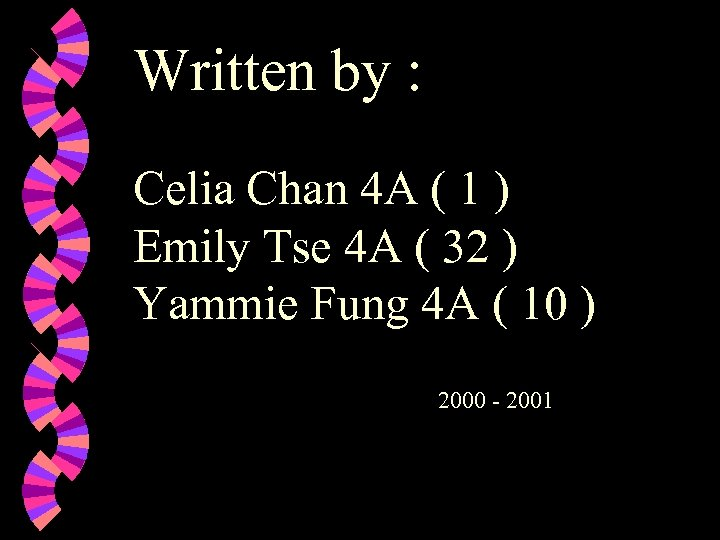Written by : Celia Chan 4 A ( 1 ) Emily Tse 4 A ( 32 ) Yammie Fung 4 A ( 10 ) 2000 - 2001